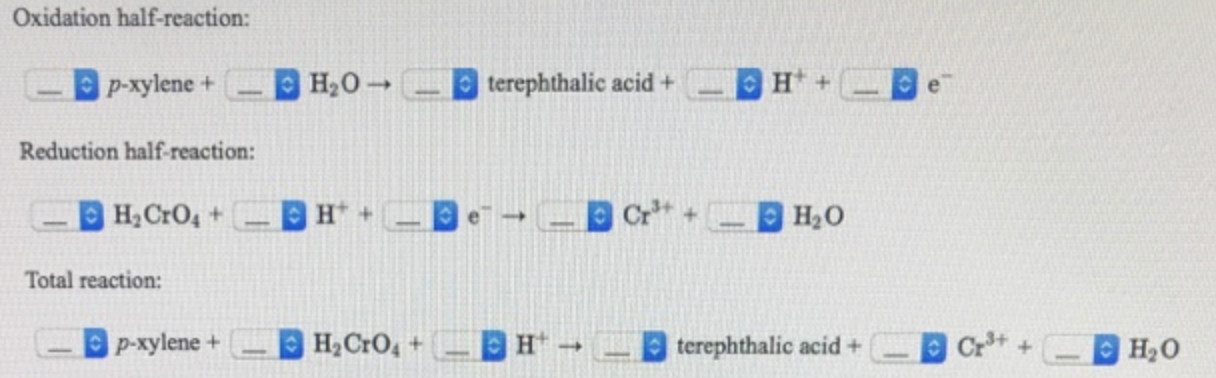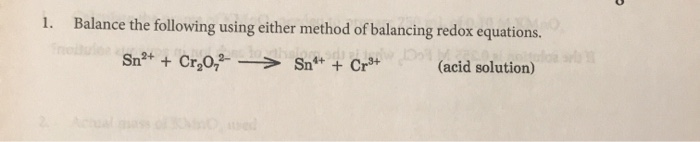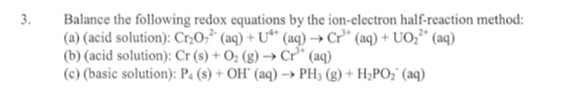Question

# balance the redox equation of: Isoborneol oxidized using Chromic Acid (H2CrO4). The products are Camphor and...

balance the redox equation of: Isoborneol oxidized using Chromic Acid (H2CrO4). The products are Camphor and Cr+3.

3 Isoborneol (-OH) + H2CrO4 + 3H+ -----------------> 3 Camphor (=O) + Cr3+ + 4H2O

Therefore,

Balanced redox reaction for oxidation of Isoborneol with H2CrO4 to Camphor is

3 Isoborneol + H2CrO4 + 3H+ -----------------> 3 Camphor + Cr3+ + 4H2O

#### Earn Coins

Coins can be redeemed for fabulous gifts.

Similar Homework Help Questions
• ### Balance the redox equation for the oxidation of p-xylene to terephthalic acid using H2CrO4:Balance the redox equation for the oxidation of p-xylene to terephthalic acid using H2CrO4: How many milligrams of H2CrO4 are required to oxidize 75.0 mg of p-xylene to terephthalic acid?

• ### 2. Balance the following redox equation using half reactions and the ion-electron method. Then...

Balance the following redox equation using half reactions and the ion-electron method. Then state what was oxidized and reduced by providing oxidationnumbers/states.MnO4- + I- ? I2 + Mn2+

• ### Complete and balance the molecular equation for the mixing of the following solutions: H2CrO4 (aq) +...

Complete and balance the molecular equation for the mixing of the following solutions: H2CrO4 (aq) + Fe(HCO3)3 (aq) -->

• ### 1. Balance the following using either method of balancing redox equations. Sn®+ Cr,0,2 -> Snº+ +...1. Balance the following using either method of balancing redox equations. Sn®+ Cr,0,2 -> Snº+ + Cr+ (acid solution)

• ### Balance the following redox equation in acid. In the blank put the correct stochiometric coefficient (0,...

Balance the following redox equation in acid. In the blank put the correct stochiometric coefficient (0, 1, 2, etc.). (Note: 0 if the species does not appear on that side of the equatio 1-SO3-(ag)+MnO4-(ag)+H+(ag)---->SO4^2-(ag)+Mn2+(ag)+H2O(l) 2- H2O2(ag)+ClO2(ag)+HO-(ag)---> O2(g)+ClO2-(ag)+H2O(l) Q: In the electrochemical voltaic cell using the reaction, Fe3+(aq) +  Cr(s) → Fe(s) + Cr3+(aq), match the correct 1/2 reaction that occurs at the anode and cathode. 1/2 reaction occuring at the anode 1/2 Reaction occuring at the cathode Choices: A Fe3+(aq) +...

• ### balance the following redox equation: Mg(s) + Cr^3+ (aq) -------> Mg^2+ (aq) + Cr(s)

balance the following redox equation: Mg(s) + Cr^3+ (aq) -------> Mg^2+ (aq) + Cr(s)

• ### Balance following equation using redox tables (acidic) (basic)

Balance following equation using redox tables (acidic) (basic)

• ### Balance the following redox half-cell reactions. Write your final equations with the oxidized species on the...

Balance the following redox half-cell reactions. Write your final equations with the oxidized species on the left hand side of the equation, and the reduced species on the right hand side of the equation. Don’t forget to pay careful attention to the stoichiometry of the various species. a) Mn2O3/MnO4- b) H2S/HSO4- c) CrO42-/Cr2O3 d) Eu2+/EuO2-

• ### 3. Balance the following redox equations by the ion-electron half-reaction method: (a) (acid solution): Cr0(aq) +...3. Balance the following redox equations by the ion-electron half-reaction method: (a) (acid solution): Cr0(aq) + U" (aq) → Cr" (aq) +UO; (aq) (b) (acid solution): Cr(s) + O2(g) → Cr* (aq) (C) (basic solution): P. (s) + OH' (aq) → PH; (g) + H2PO2 (aq)

• ### Balance the following redox reaction equation first in acidic solution and then in a basic solution: Cr (s) + MnO2 (s) -...

Balance the following redox reaction equation first in acidic solution and then in a basic solution: Cr (s) + MnO2 (s) --> Mn+2 (aq) + Cr+3 (aq)